Next: Spin Space Up: Spin Angular Momentum Previous: Introduction

# Spin Operators

Since spin is a type of angular momentum, it is reasonable to suppose that it possesses similar properties to orbital angular momentum. Thus, by analogy with Sect. 8.2, we would expect to be able to define three operators--,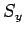, and--which represent the three Cartesian components of spin angular momentum. Moreover, it is plausible that these operators possess analogous commutation relations to the three corresponding orbital angular momentum operators,,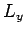, and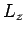[see Eqs. (531)-(533)]. In other words,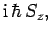(702)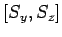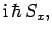(703)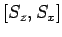(704)

We can represent the magnitude squared of the spin angular momentum vector by the operator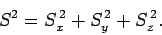(705)

By analogy with the analysis in Sect. 8.2, it is easily demonstrated that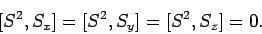(706)

We thus conclude (see Sect. 4.10) that we can simultaneously measure the magnitude squared of the spin angular momentum vector, together with, at most, one Cartesian component. By convention, we shall always choose to measure the-component,.

By analogy with Eq. (538), we can define raising and lowering operators for spin angular momentum: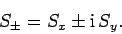(707)

If,, andare Hermitian operators, as must be the case if they are to represent physical quantities, thenare the Hermitian conjugates of one another: i.e.,(708)

Finally, by analogy with Sect. 8.2, it is easily demonstrated that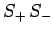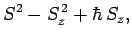(709)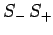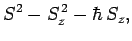(710)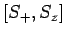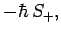(711)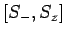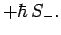(712)Next: Spin Space Up: Spin Angular Momentum Previous: Introduction
Richard Fitzpatrick 2010-07-20